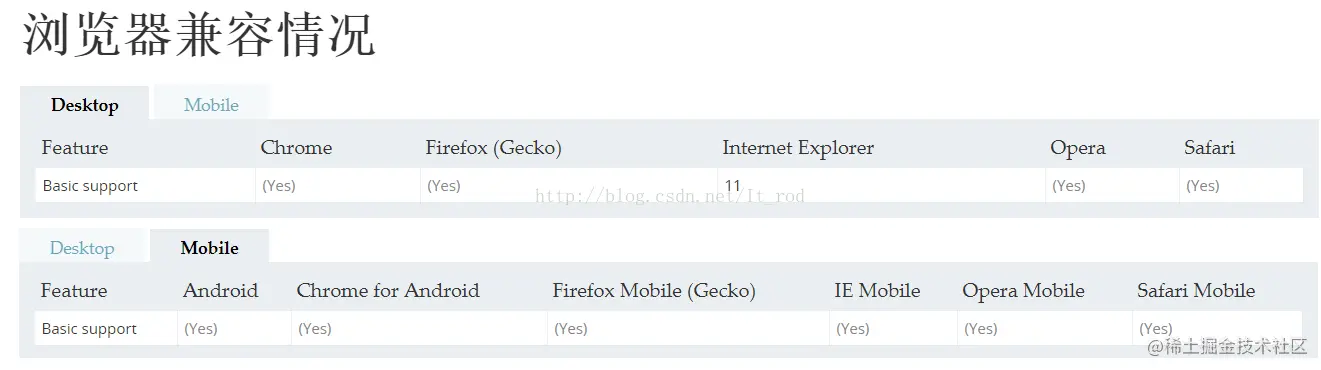1473

# 1. 构造函数模式

## (1) JS中创建新对象的三种常用方法

``````var newObject = {};

var newObject = Object.create( Object.prototype );

var newObject = new Object();

//上面的三中方式创建出来的新对象等同，Object.create(null)是一个简单的对象，不具有其他任何的属性。
``````

## (2) 创建新属性的四中方式

``````newObject.someKey = "Hello World";

newObject["someKey"] = "Hello World";

Object.defineProperty( newObject, "someKey", {
value: "for more control of the property's behavior",
writable: true,
enumerable: true,
configurable: true
});

Object.defineProperties( newObject, {
"someKey": {
value: "Hello World",
writable: true
},

"anotherKey": {
value: "Foo bar",
writable: false
}

});
``````

## (3) 用法

### 普通方式（最实用）

``````function Car(model, year, miles) {
this.model = model;
this.year = year;
this.miles = miles;
this.output= function () {
return this.model + "走了" + this.miles + "公里";
};
}

var car_benchi = new Car('benchi', '2017', '300');
var car_aodi = new Car('aodi', '2016', '600');

console.log(car_benchi.output === car_benchi.output); //false
``````

``````function Car(model, year, miles) {
var object = Object.create(null);

/*prototype*/
Object.defineProperty( object, "__proto__", {
value: Car.prototype,
writable: true,
enumerable: false,
configurable: false
})

object.model = model;
object.year = year;
object.miles = miles;

object.output = function () {
return function () {
return this.model + "走了" + this.miles + "公里";
}.call(this);
}

return object;
}

var car_benchi =  Car('benchi', '2017', '300');
var car_aodi =  Car('aodi', '2016', '600');

console.log(car_benchi.output === car_benchi.output); //false
``````

Note： 开发过程中一般不允许操作__proto__的，尽管现在大部分浏览器引起都已经支持。### prototype

``````function Car(model, year, miles) {
this.model = model;
this.year = year;
this.miles = miles;
}

Car.prototype.output= function () {
return this.model + "走了" + this.miles + "公里";
};

var car_benchi = new Car('benchi', '2017', '300');
var car_aodi = new Car('aodi', '2016', '600');

console.log(car_aodi.output === car_benchi.output); //true
``````

# 2. 工厂模式

``````function Car(model, year, miles, version) {
function carBasicInformation() {
var object = Object.create(null);

/*prototype*/
Object.defineProperty( object, "__proto__", {
value: Car.prototype,
writable: true,
enumerable: false,
configurable: false
})

object.model = model;
object.year = year;
object.miles = miles;

object.output = function () {
return function () {
return this.model + "走了" + this.miles + "公里";
}.call(this);
}

return object;
}

//0001型号有导航系统
function Car_0001 () {
var car_0001 = carBasicInformation(model, year, miles);
car_0001.omnirange = 'omnirange_01';

return car_0001;
}

//0002型号没有导航系统
function Car_0002 () {
var car_0002 = carBasicInformation(model, year, miles);

return car_0002;
}

switch (version) {
case '0001':
return Car_0001();
case '0002':
return Car_0002();
default:
break; //当然工厂肯定不会什么都不做。
}
}

var aodi_0001 =  Car('benchi', '2017', '300', '0001');
var aodi_0002 =  Car('aodi', '2016', '600', '0002');

console.log(aodi_0001.omnirange); //omnirange_01
console.log(aodi_0002.omnirange); //undeinfed
``````

# 3. 抽象工厂模式

``````//求同
function basicCarInformation(model, year, miles) { //这个地方其实可以不用这么复杂，只是本人太懒，直接将上面的代码copy了。
var object = Object.create(null);

/*prototype*/
Object.defineProperty( object, "__proto__", {
value: basicCarInformation.prototype,
writable: true,
enumerable: false,
configurable: false
})

object.model = model;
object.year = year;
object.miles = miles;

object.output = function () {
return function () {
return this.model + "走了" + this.miles + "公里";
}.call(this);
}

return object;
}

inheritAbstactFactory.prototype.basicCarInformation = basicCarInformation;

//存异
function inheritAbstactFactory(option, version) {
return function(model, year, miles) {
var basicInformation = basicCarInformation(model, year, miles);
basicInformation.option = option;
basicInformation.version = version;

return basicInformation;
}
}

var CarFactory0001 = inheritAbstactFactory({omnirange : 'omnirange_01'}, 'aodi_0001');

var aodi_0001 =  CarFactory0001('benchi', '2017', '300');
aodi_0001.output();
``````

# 4. 抽象工厂的应用

（摘抄与 design pattern）当应用于以下情况时，工厂模式可能特别有用：

• 当我们的对象或组件设置涉及高度的复杂性时
• 当我们需要根据我们所处的环境轻松生成不同的对象实例时
• 当我们正在处理许多共享相同属性的小对象或组件时
• 使用其他对象的实例组合对象时，只需要满足API合约（又名 duck typing）的工作。这对于解耦是有用的。

• 高度的负复杂性指的就是上面的逻辑判断
• 需求的变化
• 求同存异，许多共享并不是全部共享，因为全部共享的话就直接构造函数模式了
• 这里的的意义是说care的只是输入输出，至于对象是否与原型链相关不在意，就好像call,apply的用法。就是一种duck typing。

•••••••••••